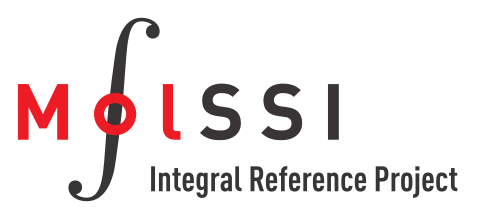MolSSI Integral Reference Project
Gaussian Product Theorem

Theory

The Gaussian Product Theorem (GPT) states that the product of two gaussians G1,G2 can be represented as a third gaussian G3 with a center a line connecting G1 and G2. This theorem is used extensively in quantum chemistry to simplify integrals.

MIRP contains functionality to compute some of the terms from the GPT which will be used within integral kernels.

Given two gaussiansand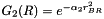centered on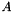and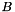, respectively, and with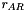andrepresenting the distance between the center of the gaussian and a given point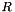Functions in MIRP

In MIRP, the parameters to the Gaussian Product Theorem can be calculated via the following functions: